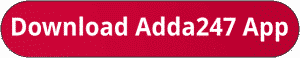Latest Banking jobs   »   Bank Foundation quant

# Quantitative Aptitude Quiz For Bank Foundation 2023 -17th July

Q1. A mixture of milk and water contains 75% milk. If 8 liter of mixture is taken out and 7 liters of milk is added, then ratio of milk to water becomes 7 : 2. Find the quantity of mixture initially.
(a) 75 L
(b) 64 L
(c) 68 L
(d) 56 L
(e) 40 L

Q2. The average weight of a class of 45 girls is 53 kg. It was later found that weight of two girls was read as 49 kg and 57 kg instead of 45 kg and 52 kg. Find the actual average weight of the class.
(a) 54 kg
(b) 53.40 kg
(c) 50.60 kg
(d) 52.80 kg
(e) 51.50 kg

Q3. The ratio of speed of boat in still water to speed of stream is 11 : 1. If the boat takes 1 hour more to cover 220 km upstream than to cover same distance in still water. Then find the speed of boat in still water.
(a) 22 km/hr
(b) 18 km/hr
(c) 15 km/hr
(d) 20 km/hr
(e) 25 km/hr

Q4. A shopkeeper in place of selling a article at 10% profit, mark up the article 30% above cost price and gives a discount of 10%. In this process he gets Rs. 56 more profit. Find the cost price of article.
(a) Rs. 400
(b) Rs. 1000
(c) Rs. 800
(d) Rs. 600
(e) Rs. 500

Q5. In how many ways the word ‘INTICINCO’ can be written so that ‘T’ always comes at end.
(a) 720
(b) 1680
(c) 5040
(d) 1024
(e) 210

Q6. A sum becomes 160% of amount at simple interest in 3 years. Find the compound interest on sum of Rs 12000 at same rate of interest after 2 years.
(a) Rs 4820
(b) Rs 5460
(c) Rs 6280
(d) Rs 5280
(e) Rs 5840

Q7. A bag contains 15 balls numbered from 1 to 15. Two balls are selected randomly without replacement. Find the probability that both balls are even number ball.
(a) 1/5
(b) 3/5
(c) 2/5
(d) 4/5
(e) 13/15

Q8. A person bought an old bicycle in Rs 6400. He expends Rs. X on repairing it. Find value of X, if he sold the bicycle in Rs 9180 and makes profit of 20%.
(a) Rs 1450
(b) Rs 1250
(c) Rs 1800
(d) Rs 1350
(e) Rs 1150

Q9. In a class of N students, a new student weighing 35 kg joined due to which average weight of the class increased by 1 kg, if the weight of new student would have be15 kg, then the average weight of the class decreases by 1 kg, then find the total no. of students in class initially.
(a) 12
(b) 7
(c) 15
(d) 11
(e) 9

Q10. Ratio of two numbers is 5 : 7. If 30 is added in each number, the ratio becomes 3 : 4. Find the ratio of the number if 10 is subtracted from each number.
(a) 5 : 8
(b) 8 : 11
(c) 7 : 10
(d) 6 : 11
(e) 7 : 9

Solutions:## FAQs

### What is the selection process of the Bank Clerk?

The selection process of the Bank Clerk is Prelims & Mains.

#### Congratulations!Union Budget 2023-24: Free PDF